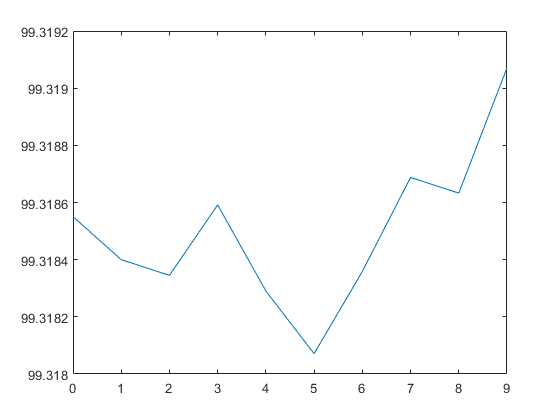# Measure Frequency Using NI Devices

This example shows how to measure frequency to determine rate of flow of fluid using a flow sensor. The sensor generates a digital signal with frequency that correlates to the rate of flow of fluid.

### Create a Counter Input Channel

Use `daq` to create a DataAcquisition and `addinput` to add a counter input channel with `Frequency` measurement type. For this example, use CompactDAQ chassis NI c9178 and module NI 9402 with ID cDAQ1Mod5.

```dq = daq("ni"); ch = addinput(dq,"cDAQ1Mod5", "ctr0", "Frequency"); ch ```
```ch = Index Type Device Channel Measurement Type Range Name _____ ____ ___________ _______ ________________ _____ ________________ 1 "ci" "cDAQ1Mod5" "ctr0" "Frequency" "n/a" "cDAQ1Mod5_ctr0" ```

### Determine the Terminal of the Counter Input Channel

To connect the input signal to the correct terminal, examine the `Terminal` property of the channel. The terminal is determined by the hardware.

```ch.Terminal ```
```ans = 'PFI1' ```

### Measure Frequency

To determine if the counter is operational, input a single scan while the motor is rotating.

```read(dq) ```
```ans = timetable Time cDAQ1Mod5_ctr0 _____ ______________ 0 sec 100 ```

### Monitor Frequency over Time

Use the hardware clock to acquire multiple counter measurements over time. NI counter devices require an external clock. By adding an analog input channel for a module on the same chassis, the session shares an internal clock with both modules.

```dq = daq("ni"); dq.Rate = 1; addinput(dq,"cDAQ1Mod1", "ai0", "Voltage"); addinput(dq,"cDAQ1Mod5", "ctr0", "Frequency"); data = read(dq, seconds(10)); plot(data.Time, data.cDAQ1Mod5_ctr0); ```##### SupportGet trial now